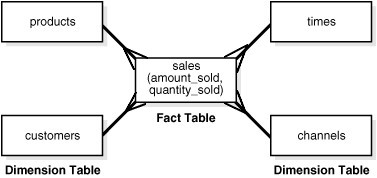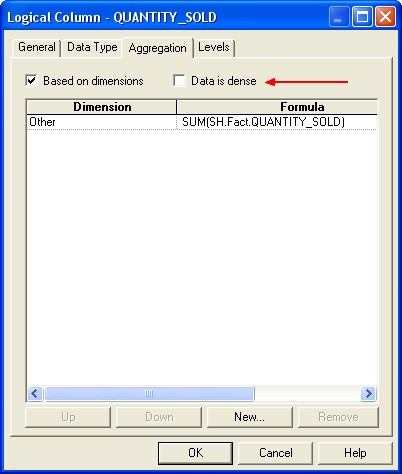# SQL Function - Window Aggregate (Analytics function)

Windowing functions (known also as analytics) allow to compute:

They are distinguished from ordinary SQL functions by the presence of an OVER clause.

With windowing aggregate functions, you can calculate moving and cumulative versions of SUM, AVERAGE, COUNT, MAX, MIN, and many more functions.

Analytic functions compute an aggregate value based on a group of rows. They differ from aggregate functions in that they return multiple rows for each group. The group of rows is called a window.

Analytic functions enable you to calculate:

• First/last analysis
• Linear regression statistics

Analytic functions can be used in the following areas where standard SQL fails to impress.

• Ranking data within subsets of a data set.
• Aggregating data within subsets of a data set.
• Performing aggregations over moving windows.
• Displaying and comparing aggregates to individual entries within a single query.
• Comparing two or more rows within a given data set.
• Fetching from the translate table the values based on EFFDT.

Partition in the clause statement define the subset.

The data property dense/parse is really important when using this functions.

Analytic functions allow to replace procedural by set based logic.

True streaming platform (not microbatch) will handles late arriving data. The window is never really closed and some late arriving data with a timestamp inside the window can arrive at any time.

## The process

Query processing using analytic functions takes place in three stages :

1. all joins, WHERE, GROUP BY and HAVING clauses are performed.
2. the result set is made available to the analytic functions, and all their calculations take place.
3. if the query has an ORDER BY clause at its end, the ORDER BY is processed to allow for precise output ordering.

## Functions

List of analytic functions

## Reference

Discover More(Function | Operator | Map | Mapping | Transformation | Method | Rule | Task | Subroutine)

Section computable function. A function is a callable unit that may be called: a procedure, a subrontine a routine, a method (belong to an objectmacrocomputablalgorithreusable blocargumentdevelopment...Analytic (Data Analyst)

A Data Analyst sees what data he has. A Data Scientist imagines what data he is lacking. Information analysis provides insight into : the past the present and the future of the business. See...Dimensional Data Modeling - Descriptif Attribute (Dimensional Attribute)

A descriptif attribute is class attribute that describe a property or characteristic of a dimension. They are used to label, filter and/or group on. measures Typical attributes for a product dimension...Function - (Moving|Rolling|Running) Calculation

(Moving|Rolling|Running) Calculation are computed with window function. For example, running total of sum (sal + comm) department wise on emp table : Oracle in Sqlplus:How to use the Aggregate / Window Functions (sum, avg, ) in Sqlite ?

... The aggregate / window function in Sqlite. Sqlite supports the following aggregate /window function : SUM (total) AVG Max Min Rank row_number more see the Specification...Number - Addition (or Sum, Sigma) or Total

The addition of all numbers in a set is called a total. Sum can be use as: an aggregate or a analytic function. It can follow the full syntax of the analytic function and in this way create It's...OBIEE - What means Data is dense ?

When setting up a aggregate rules for a measure column, you can specify that data is dense only if all the logical table sources to which it is mapped are dense. In a logical column if you check “Based...Oracle Database - SQL - Analytic Functions

Analytic functions in the Oracle Database Context An analytic function takes place after that the original data set is retrieved. The clause AVG CORR COVAR_POP COVAR_SAMP...Oracle Database - SQL - First Value Analytic function

FIRST_VALUE is an non-deterministic analytic function. It returns the first value in an ordered set of values. If the first value in the set is null, then the function returns NULL unless you specify...Ordinal Data - Rank function (Ranking)

Rank is a statistic / function that calculates the rank of a value in a group of values. The position of an element in a sequence is its rank or index. This function is used in: top-N and bottom-N...